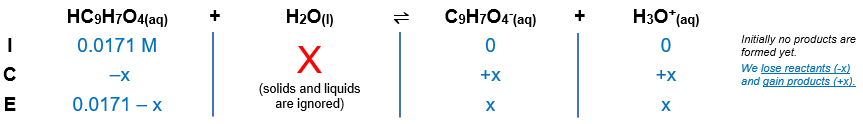# Problem: The active ingredient in aspirin is acetylsalicylic acid (HC9H7O4), a monoprotic acid with a Ka of 3.3x10-4 at 25°C .What is the pH of a solution obtained by dissolving two extra-strength aspirin tablets, containing 510 mg of acetylsalicylic acid each, in 330 mL of water?

###### FREE Expert Solution

Equilibrium reaction:        HC9H7O4(aq) + H2O(l)  C9H7O4-(aq) + H3O+(aq)

Step 1: Calculate the concentration of the solution.

molar mass HC9H7O4 = 180.154 g/mol

mass HC9H7O4 = 510 mg x 2 = 1020 mg

moles HC9H7O4 = 5.6618x10–3 mol

[HC9H7O4] = 0.0171 M

Step 2: Construct an ICE chart for the reaction.Step 3: Write the Ka expression.

${\mathbf{K}}_{{\mathbf{a}}}\mathbf{=}\frac{\mathbf{products}}{\mathbf{reactants}}\phantom{\rule{0ex}{0ex}}\overline{){\mathbf{K}}_{\mathbf{a}}\mathbf{=}\frac{\mathbf{\left[}{\mathbf{C}}_{\mathbf{9}}{\mathbf{H}}_{\mathbf{7}}{{\mathbf{O}}_{\mathbf{4}}}^{\mathbf{-}}\mathbf{\right]}\mathbf{\left[}{\mathbf{H}}_{\mathbf{3}}{\mathbf{O}}^{\mathbf{+}}\mathbf{\right]}}{\left[{\mathrm{HC}}_{9}{H}_{7}{O}_{4}\right]}}$

Solids and liquids are not included in the expression

Step 4: Calculate the equilibrium concentrations.

${\mathbf{K}}_{\mathbf{a}}\mathbf{=}\frac{\left[\mathbf{x}\right]\left[\mathbf{x}\right]}{\left[\mathbf{0}\mathbf{.}\mathbf{0171}\mathbf{-}\mathbf{x}\right]}\phantom{\rule{0ex}{0ex}}\mathbf{3}\mathbf{.}\mathbf{3}\mathbf{×}{\mathbf{10}}^{\mathbf{-}\mathbf{4}}\mathbf{=}\frac{{\mathbf{x}}^{\mathbf{2}}}{\mathbf{0}\mathbf{.}\mathbf{0171}\mathbf{-}\mathbf{x}}$

$\frac{{\left[{\mathrm{HC}}_{9}{H}_{7}{O}_{4}\right]}_{\mathbf{initial}}}{{\mathbf{K}}_{\mathbf{a}}}\mathbf{\ll }\mathbf{500}$

$\overline{){\mathbf{x}}{\mathbf{=}}\frac{\mathbf{-}\mathbf{b}\mathbf{±}\sqrt{{\mathbf{b}}^{\mathbf{2}}\mathbf{-}\mathbf{4}\mathbf{a}\mathbf{c}}}{\mathbf{2}\mathbf{a}}}$

92% (251 ratings)###### Problem Details

The active ingredient in aspirin is acetylsalicylic acid (HC9H7O4), a monoprotic acid with a Ka of 3.3x10-4 at 25°C .

What is the pH of a solution obtained by dissolving two extra-strength aspirin tablets, containing 510 mg of acetylsalicylic acid each, in 330 mL of water?